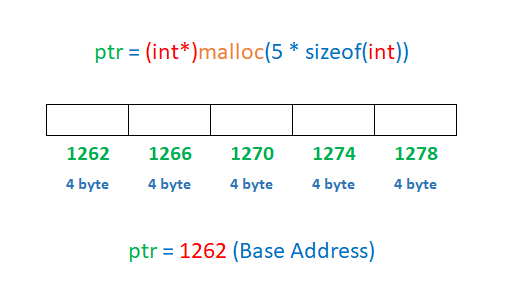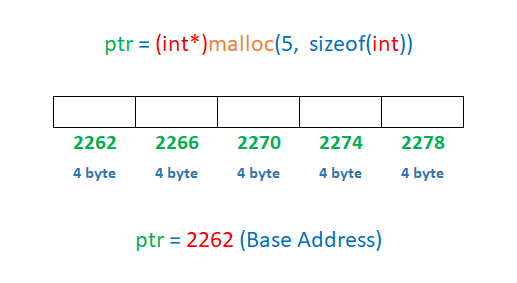# Dynamic Memory Allocation in C Programming

###### Pointer and Structure in C

In this lesson, we will understand what is Dynamic Memory Allocation in C Programming along with some examples.

## What is Dynamic Memory Allocation in C

The process of allocating memory during runtime (execution of the program) is known as Dynamic Memory Allocation.

In C programming, the allocating and releasing of memory space is done, with the use of built-in functions like sizeof(), malloc(), calloc(), realloc() and free(). To use these functions, we need to include malloc.h header file in our program.

Let's learn all these functions in detail.## sizeof() Function

The sizeof() function returns the size of its argument in terms of bytes. The argument can be a variable, array, structure or any data type like int, float, double char etc.

### Syntax of sizeof() Function

``sizeof(argument)``

#### Example

C program to show the use of sizeof() function.

``````#include <stdio.h>
#include <conio.h>

int main()
{
int a=86;
float b[]= {15,69,74,82};
double c=47.2369;
char d[]="Hello World";

printf("Size of int = %d bytes\n",sizeof(int));
printf("Size of float = %d bytes\n",sizeof(float));
printf("Size of double = %d bytes\n",sizeof(double));
printf("Size of char = %d byte\n\n",sizeof(char));

printf("Size allocate for variable a = %d bytes\n",sizeof(a));
printf("Size allocate for variable b = %d bytes\n",sizeof(b));
printf("Size allocate for variable c = %d bytes\n",sizeof(c));
printf("Size allocate for variable d = %d bytes\n",sizeof(d));
return 0;
}``````

#### Output

```Size of int = 4 bytes
Size of float = 4 bytes
Size of double = 8 bytes
Size of char = 1 byte

Size allocate for variable a = 4 bytes
Size allocate for variable b = 16 bytes
Size allocate for variable c = 8 bytes
Size allocate for variable d = 12 bytes```

We can see that sizeof() function returns the size of int=4 bytes, float=4 bytes, double=8 bytes and char=1 byte.

The size allocated for int type variable a is 4 bytes.

The size allocated for float type array b is 16 bytes because each element of the float type array occupies 4 bytes of memory space. As there are 4 elements in the array, so it will take 4*4=16 bytes space in the memory.

The size allocated for double type variable c is 8 bytes.

The size allocated for char type array d is 12 bytes because each element of the char type array occupies 1 byte of memory space. As there are 12 characters in the array including space and null character, so it will take 12 bytes space in the memory.

## malloc() Function

The malloc() function is used to allocate space in the memory during runtime (execution of the program). This function allocates a memory space of specified size and return the starting address to pointer variable.

### Syntax of malloc() Function

``pointer_variable = (datatype*)malloc(specified_size);``

#### Example 1

``````#include <stdio.h>
#include <conio.h>
#include <malloc.h>

int main()
{
int *ptr;
ptr=(int*)malloc(sizeof(int));
return 0;
}``````

Here ptr is an integer type pointer variable that holds the memory address allocated by malloc() function. (int*) means that the function will allocate memory to store an integer type value. malloc(sizeof(int)) allocates memory space of integer size (4 bytes).

#### Example 2

``````#include <stdio.h>
#include <conio.h>
#include <malloc.h>

int main()
{
int *ptr;
ptr=(int*)malloc(5 * sizeof(int));
return 0;
}``````

Here we allocate the memory space for 5 integers, and the base address is stored in the pointer variable ptr. See the example image given below.#### Example 3

C program to store and display 5 integer numbers by dynamic memory allocation using malloc() function.

``````#include <stdio.h>
#include <conio.h>
#include <malloc.h>

int main()
{
int *ptr,i;
ptr=(int*)malloc(5 * sizeof(int));

printf("Enter 5 numbers\n");
for(i=0; i<5; i++)
{
scanf("%d",(ptr+i));
}

for(i=0; i<5; i++)
{
printf("%d ",*(ptr+i));
}
return 0;
}``````

#### Output

```Enter 5 numbers
12
89
47
64
23
12 89 47 64 23```

In the above program, we are accessing the consecutive memory addresses by adding the value of i to the base address stored in ptr.

## calloc() Function

The calloc() function is also used to allocate space in the memory during runtime (execution of the program) like the malloc() function but, the calloc() function requires two arguments. The first argument is the number of blocks and, the second argument is the size per block. After allocating the memory blocks, it returns the address of the first block.

### Syntax of calloc() Function

``pointer_variable = (datatype*)calloc(number_of_blocks, size_per_block);``

#### Example 1

``````#include <stdio.h>
#include <conio.h>
#include <malloc.h>

int main()
{
int *ptr;
ptr=(int*)calloc(5, sizeof(int));
return 0;
}``````

In the above program, we have allocated 5 integer blocks of memory to store 5 integer numbers. The base address of the first block is stored in pointer variable ptr. See the example image given below.#### Example 2

C program to store and display 5 integer numbers by dynamic memory allocation using calloc() function.

``````#include <stdio.h>
#include <conio.h>
#include <malloc.h>

int main()
{
int *ptr,i;
ptr=(int*)calloc(5, sizeof(int));

printf("Enter 5 numbers\n");
for(i=0; i<5; i++)
{
scanf("%d",(ptr+i));
}

for(i=0; i<5; i++)
{
printf("%d ",*(ptr+i));
}
return 0;
}``````

#### Output

```Enter 5 numbers
8
63
49
15
32
8 63 49 15 32```

The one major difference between malloc() and calloc() is that the malloc() function allocates memory but does not initialize it with a default value and, space contains garbage value after allocation. The calloc() function allocate space and initialize it with the default value 0 and, space does not contains garbage value after allocation .

#### Example

``````#include <stdio.h>
#include <conio.h>
#include <malloc.h>

int main()
{
int *ptr1,*ptr2;
ptr1=(int*)malloc(sizeof(int));
ptr2=(int*)calloc(1,sizeof(int));

printf("ptr1 points to value %d\n", *ptr1);
printf("ptr2 points to value %d\n", *ptr2);
return 0;
}``````

#### Output

```ptr1 points to value 1840480
ptr2 points to value 0```

Here 1840480 is a garbage value and it may change each time when we run the program.

## realloc() Function

The realloc() function is used to increase or decrease size of the allocated memory at runtime. The realloc() function only works on dynamically allocated memory. If the memory is not allocated dynamically using malloc() or calloc(), then the behavior of the realloc() function is undefined.

### Syntax of realloc() Function

``pointer_variable = (datatype*)realloc(pointer_variable, new_size);``

#### Example 1 (Increase memory size at runtime)

``````#include <stdio.h>
#include <conio.h>
#include <malloc.h>

int main()
{
int *ptr,i;

// Allocate memory to store 2 integers
ptr=(int*)calloc(2,sizeof(int));

*(ptr)=10;
*(ptr+1)=20;

// Increase memory to store 3 integers
ptr=(int*)realloc(ptr, 3*sizeof(int));
*(ptr+2)=30;

for(i=0; i<3; i++)
{
printf("%d ",*(ptr+i));
}
return 0;
}``````

#### Output

`10 20 30`

#### Example 2 (Decrease memory size at runtime)

``````#include <stdio.h>
#include <conio.h>
#include <malloc.h>

int main()
{
int *ptr,i;

// Allocate memory to store 3 integers
ptr=(int*)calloc(3,sizeof(int));

// Decrease memory to store only 2 integers
ptr=(int*)realloc(ptr, 2*sizeof(int));
return 0;
}``````

## free() Function

The free() function is used to release the memory, which is dynamically allocated by the functions malloc() or calloc().

### Syntax of free() Function

``free(pointer_variable);``

#### Example

``````#include <stdio.h>
#include <conio.h>
#include <malloc.h>

int main()
{
int *ptr;

// Allocate memory to store 1 integer
ptr=(int*)malloc(sizeof(int));

// Release the allocated memory
free(ptr);
return 0;
}``````

Note: For efficient use of memory, it is important to release the allocated space using the free() function when the allocated space is no more required in the program.